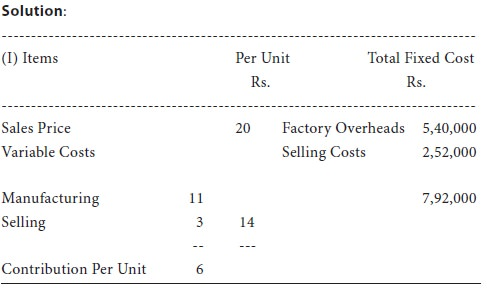Home | ARTS | Accounting For Managers | Application Of Cost-Volume-Profit Analysis

# Application Of Cost-Volume-Profit Analysis

Posted On :  03.05.2018 10:08 pm

Application Of Cost-Volume-Profit Analysis

Application Of Cost-Volume-Profit Analysis

CPV analysis helps in:

ՖՖ                     Forecasting the profit in an accurate manner

ՖՖ                     Preparing the flexible budgets at different levels of activity

ՖՖ                     Fixing prices for products

## Illustration 1:

(Profit Planning) based on the following information, find out the break even point, the sales needed for a profit of rs.6,00,000 and the profit if 4,00,000 units are sold at rs.6 per unit.

Units Of Output                                                   5,00,000

Fixed Costs                                                    Rs.7,50,000

Variable Cost Per Unit                                    Rs. 2

Selling Price Per Unit                                     Rs. 5## Illustration 2: (Pricing)

A company is considering a reduction in the price of its product by 10% because it is felt that such a step may lead to a greater volume of sales. It is anticipated that there will be no change in total fixed costs or variable costs per unit. The directors wish to maintain profit at the present level.

You are given the following information:

 Sales (15,000 Units) Rs.3,00,000 Variable Cost Rs.13 Per Unit Fixed Cost Rs.60,000

From the above information, calculate P/V ratio and the amount of sales required to maintain profit at the present level after reduction of selling price by 10%.## Illustration 3:

From the following data, calculate the break-even point.

First year                            Second Year

Sales                                        80,000                               90,000

Profit                                   Rs.10,000                          Rs.14,000## Illustration 4:

A company is considering expansion. Fixed costs amount to rs.4,20,000 and are expected to increase by rs.1,25,000 when plant expansion is completed. The present plant capacity is 80,000 units a year. Capacity will increase by 50 percent with the expansion. Variable costs are currently rs.6.80 per unit and are expected to go down by re.0.40 per unit with the expansion. The current selling price is rs.16 per unit and is expected to remain the same under either alternative. What are the break-even points under either alternatives? Which alternative is better and why?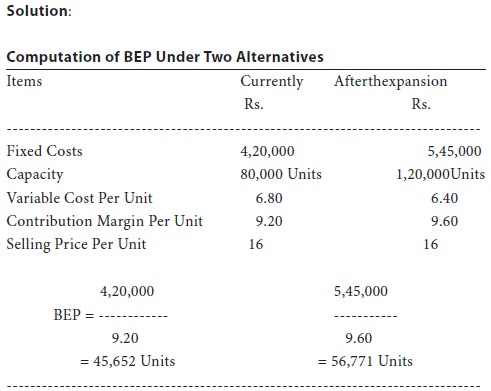Assuming that the whole production can be sold, the profit under

The two alternatives will be:It is obvious from the above calculations that the profits will be almost double after the expansion. Hence, the alternative of expansion is to be preferred.

## Illustration 5:

A factory engaged in manufacturing plastic buckets is working at 40% capacity and produces 10,000 buckets per annum:
 Rs. Material 10 Labour cost 3 Overheads 5 (60% fixed)

The selling price is rs.20 per bucket.

If it is decided to work the factory at 50% capacity, the selling price falls by 3%. At 90% capacity the selling price falls by 5%, accompanied by a similar fall in the prices of material.
You are required to calculate the profit at 50% and 90% capacities and also the break-even points for the same capacity productions.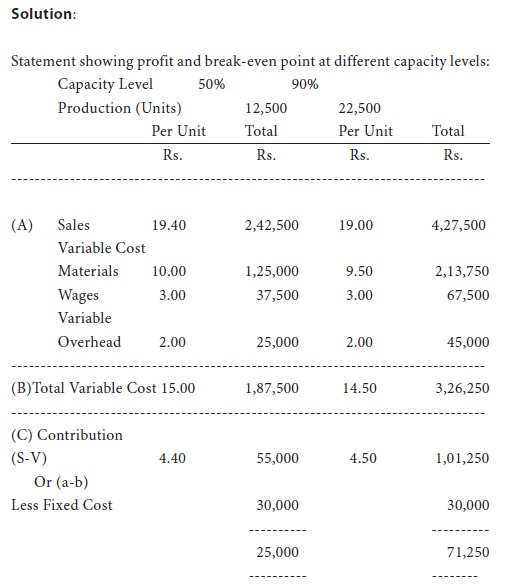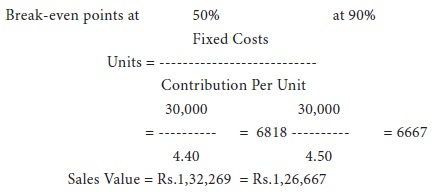## Illustration 6:

Calculate:

ՖՖ The amount of fixed expenses

ՖՖ The number of units to break-even

ՖՖThe number of units to earn a profit of rs.40,000 The selling price can be assumed as rs.10.

The company sold in two successive periods 9,000 units and 7,000 units and has incurred a loss of rs.10,000 and earned rs.10,000 as profit respectively.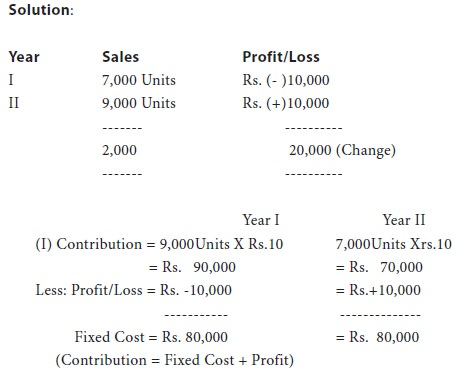## Illustration 7:

From The Following Data Calculate:

ՖՖ                    P/V Ratio

ՖՖ                    Profit When Sales Are Rs.20,000

ՖՖ             Net Break-Even If Selling Price Is Reduced By 20%

Fixed Expenses Rs.4,000

Break-Even Point 10,000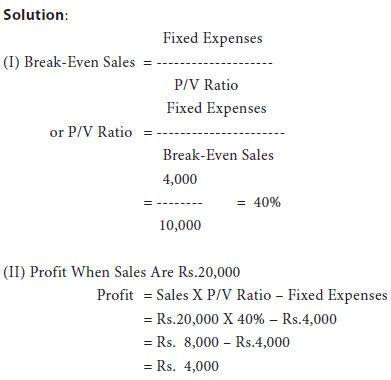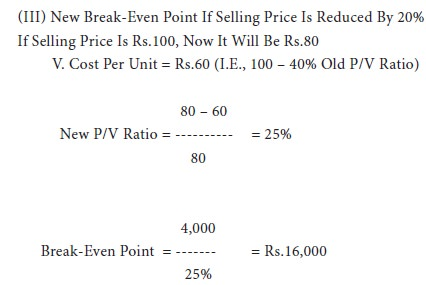## Illustration 8:

From the following data calculate:

ՖՖ            Break-even point in amount of sales in rupees.

ՖՖ            Number of units that must be sold to earn a profit of Rs.60,000 Per year.

ՖՖ            How many units must be sold to earn a net profit of 15% of sales?

Sales Price                                                       Rs.20 Per Unit

Variable manufacturing costs                             Rs.11 per unit

Variable selling costs                                         Rs. 3 per unit